## Most Used Formulas (cont.)

 Q or Figure of Merit Impedance (cont.) of a simple reactor In series circuits where phase angle and any two of the Z, R and X components are known, the unknown component may be determined from the expressions: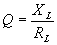of a single capacitor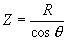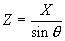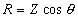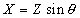where Q  = a ratio expressing the figure of  merit, where Z  = magnitude of impedance in ohms, XL  = inductive reactance in ohms, R  = resistance in ohms, XC  = capacitive reactance in ohms, X  = reactance (inductive or  capacitive) in ohms, RL  = resistance in ohms acting in series  with inductance, Nomenclature RC  = resistance in ohms acting in series  with capacitance, Z  = absolute or numerical value of  impedance magnitude in ohms, Impedance R  = resistance in ohms, In any a-c circuit where resistance and reactance values of the R, L and C components are given, the absolute or numerical magnitude of impedance and phase angle can be computed from the formulas which follow: XL  = inductive reactance in ohms, XC  = capacitive reactance in ohms, L  = inductance in henrys, C  = capacitance in farads, In general the basic formulas expressing total impedance are: RL  = resistance in ohms acting in  series with inductance, for series circuits, RC  = resistance in ohms acting in  series with capacitance,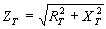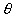= phase angle in degrees by which  current leads voltage in a capacitive  circuit, or lags voltage in an inductive  circuit.  In a resonant circuit , where XL equals XC,equals 0o. for parallel circuits,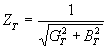Degrees X 0.0175 = radians.  1 radian = 57.3o See page 17 for formulas involving impedance, conductance, susceptance and admittance. Numerical Magnitude of Impedance . . .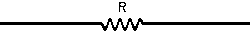of resistance alone Z = R= 0o